Showing posts with label ideas. Show all posts
Showing posts with label ideas. Show all posts

May 2, 2019

Making it up the mountain

I've been making my way up the mountain. That's both real and metaphorical.

The walk up Beacon mountain starts just three houses from mine. I've climbed to the top a bunch of times, but lately it seems like more of a struggle.

Today I'm happy to say I made it even further up.
The bugs getting stuck in my hair were actually more of a challenge than the steep climb. Which reminded me of course of a song my father used to sing to me. Because most things in life remind me of a song my father used to sing to me.

Hair from the play Hair:
"Darlin', give me a head with hair, long beautiful hair. Shining, gleaming, steaming, flaxen, waxen. Give me down to there hair, shoulder length or longer. Hair, hair, hair, hair, hair, hair, hair, hair. I want it long, straight, curly, fuzzy, snaggy, shaggy, ratty, Matty, oily, greasy, fleecy, shining, gleaming, streaming, flaxen, waxen. Hair, hair, hair, hair."

I have this idea to make a book of photocopies of my hair. Here are the first two.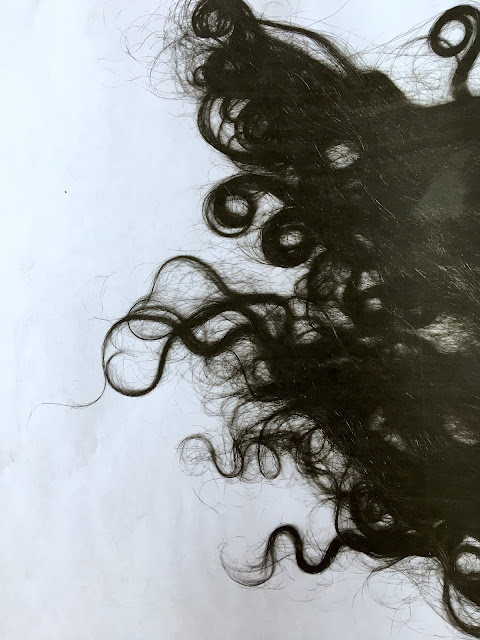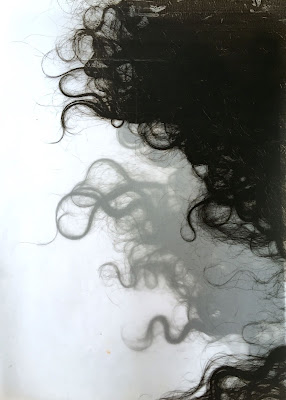March 19, 2019

IBS: An Exhibition Proposal

I n s i d e   a n d   O u t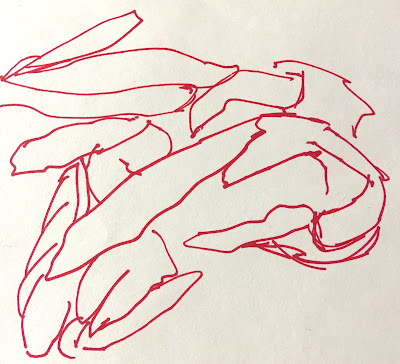Samantha Palmeri, untitled drawing

I   a m   s o   s l o w ,   i n s i d e   a n d   o u t .   I ' m   s l o w   t o   a b s o r b   a n d   r e c o g n i z e ,   s l o w   t o   p r o c e s s ,   a n d   s l o w   t o   a c c e p t .   I ' m   s o   s l o w   i t ' s   t a k e n   m e   4 5   y e a r s   t o   r e a l i z e   h o w   s l o w   I   a m .   I t ' s   t a k e n   m e   h a l f   a   l i f e t i m e   t o   c o n n e c t   t h e       d o t s   i n   m y   l i f e ,   s o m e   t h a t   a r e   p r o b a b l y   v e r y   o b v i o u s   t o   a n y o n e   l o o k i n g .   T h i n g s   t h a t   h a p p e n   i n   c h i  l d h o o d   o f t e n   b e c o m e   t h i n g s   t h a t   y o u   m a k e   h a p p e n   i n   a d u l t h o o d .   I r o n i c a l l y   I ' m   a   v e r y   i m p a t i e n t   p e r s o n   w h e n   i t     c o m e s   t o   m y   f e e l i n g s ,   a n d   t h i s   t o r t o i s e   l i k e   s l o w   p r o c e s s   d o e s   n o t  b o d e   w e l l   w i t h   m y   u r g e n c y .

E v e n   m y   b o d y   i s   s l o w .   I   i n t e r n a l i z e   e v e r y t h i n g .  I   d o n ' t   t h i n k   i t ' s   i r o n i c   t h a t   I   h a v e   d i g e s t i o n   p r o b l e m s .   Y o u   c a n   o n l y   s w a l l o w   d o w n   s o   m u c h   b e f o r e   t h i n g s   g e t   t e r r i b l y   b a c k e d   u p .  I ' m   l i k e   a   d u m p  t r u c k  t h a t  g e t s   f i l l e d   a n d   e m p t i e d ,   f i l l e d   a n d   e m p t i e d .

T a k e   t h e   d i g e s t i v e   s y s t e m .   Y o u   i n g e s t   f o o d ,   y o u r   b o d y   d i g e s t s   i t ,   a n d   i t   c o m e s   o u t   o f   y o u .  F o r   p e o p l e   w i t h   I B S   l i k e   m e ,  t h a t   s y s t e m   d o e s n ' t  w o r k   p r o p e r l y . I n   s o m e   c a s e s   y o u   i n g e s t   f o o d ,   y o u r   b o d y   d o e s n ' t   d i g e s t   i t ,   a n d   i t   s t a y s   f o r   t o o   l o n g   u n t i l   i t   f i n a l l y   e x p l o d e s   o u t   o f   y o u .     M y   h u s b a n d   h a s   t o l d   m e   t h i s   i s   a   l o t   l i k e   m y   p e r s o n a l i t y ,   t h a t  I  b o t t l e   t h i n g s   u p   u n t i l   I   e x p l o d e ,   u s u a l l y   w h e n   w e   l e a s t   e x p e c t   i t .

M y   p h y s i c a l   b o d y   s e e m s   t o   b e   m a n i f e s t i n g   t h e   i n v i s i b l e   f o r c e s   t h a t     m a k e   u p   m y   h e a r t   a n d   m i n d .

I ' v e   b e e n   t h i n k i n g   a b o u t   a l l   t h e s e   t h i n g s   l i k e   m a y b e   t h e r e ' s   a n   e x h i b i t i o n   h e r e .   M a y b e   t h i s   i s   a   g o o d   s t o r y   f o r   a   n e w   s e r i e s    o f   p a i n t i n g s .      T h e   s e r i e s   c o u l d   b e   a l l   v e r y   l a r g e   d i p t y c h s   t o   r e p r e s e n t   t h e   d u a l i t y     o f   v e r y   l a r g e   h a l f   d i g e s t e d   t r u t h s .   P e r h a p s   t h e r e   w o u l d   b e   l o t s   o f   i r o n y .   A n d   i t   w o u l d   b e  t i t l e d   I n s i d e   a n d   O u t .

March 10, 2014

How Much Of The Audience Should I Be Concerned With?

This is a repost of something I wrote back in 2014. It's crazy that I just stumbled across it and it's like I could've just written it yesterday!

Thankfully I feel like the new series of paintings I'm working on is resolving this very issue. I guess we'll just have to wait another 3 years to see if it still applies!

.................................................................................................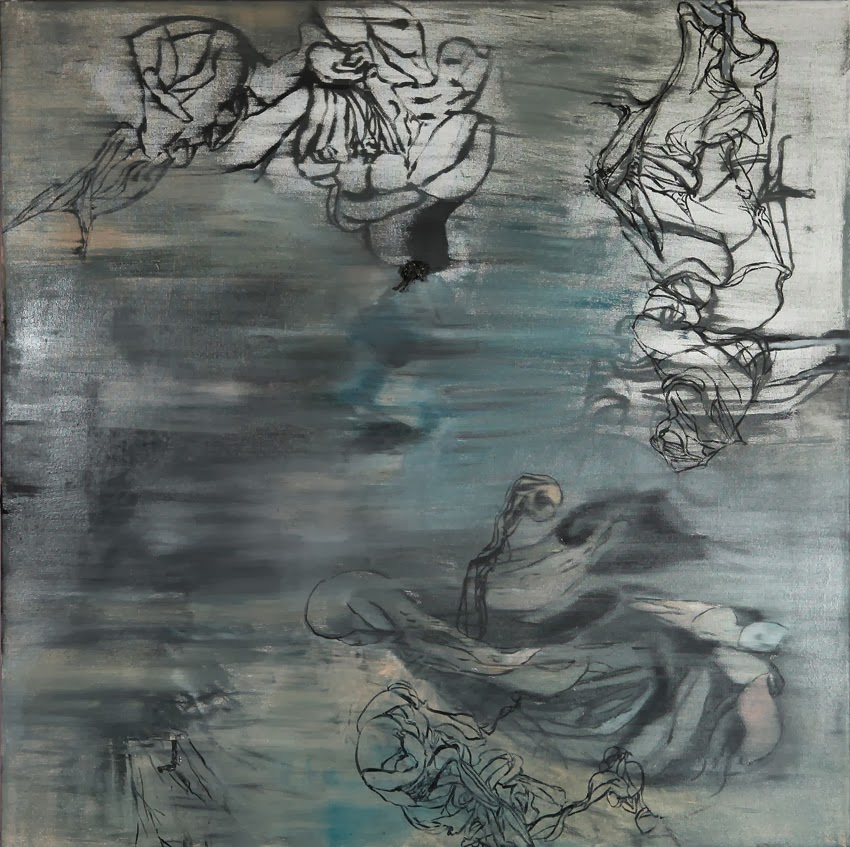laundry meat after a funeral, 2011, 44X44", oil on canvascaress, 2009, oil on canvas, 54X56"

March 2014

I've always been interested in the figure, but not necessarily in figure painting. I prefer a blurry line between the figurative and abstract. I'd much rather offer a question to the viewer than a declaration.

Although it's been a while since I made the more definitive transition to pure abstraction, lately I've been looking at some of my older figurative work with a discerning eye.

I notice a big difference in the way people respond to the recognizable versus the unrecognizable. And now that I'm thinking about it, I notice a big difference in the way I'm responding myself. It's like there was more to look at before, more of an essence.

Most viewers had a much stronger reaction to the work that was more recognizable. I thought it was just that figures and faces were more familiar. It's also hard to experience abstract work when you're spending the whole time trying to 'figure it out' instead of actually looking, which is what people tend to do.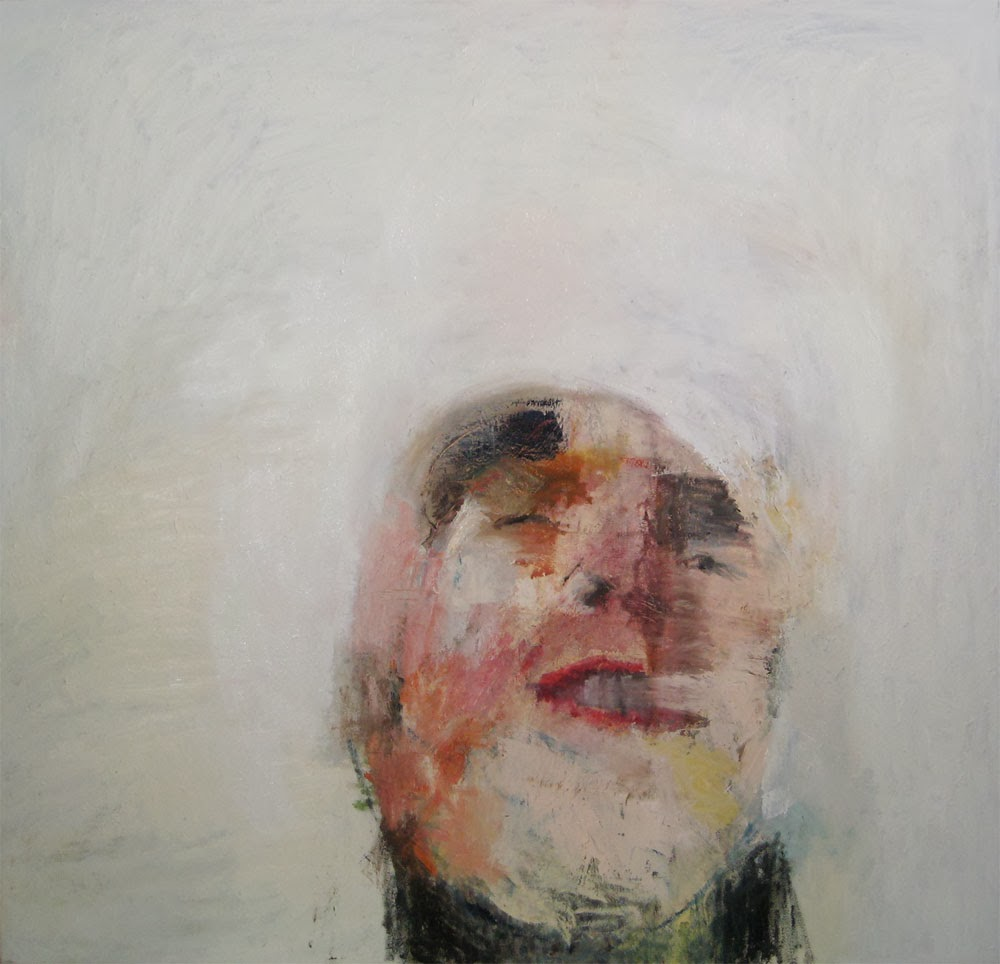ugly head, 2009, oil on canvas, 54X54"detail, in like a lion, 2011, oil on canvas, 50X76"
These paintings have a lot in common, but I do feel that there is something almost tangible in the figures that is missing in the abstractions. If I could just get that thing into the abstract paintings...

It's that human connection I've been searching for in all my work, but perhaps it was clearer to a broader audience before.

I wonder, how much of the audience should I be concerned with?

What do you think?the new swimmer, 2009, triptych, 178X50", oil on canvasskinny, 2012, oil on canvas, 30X50"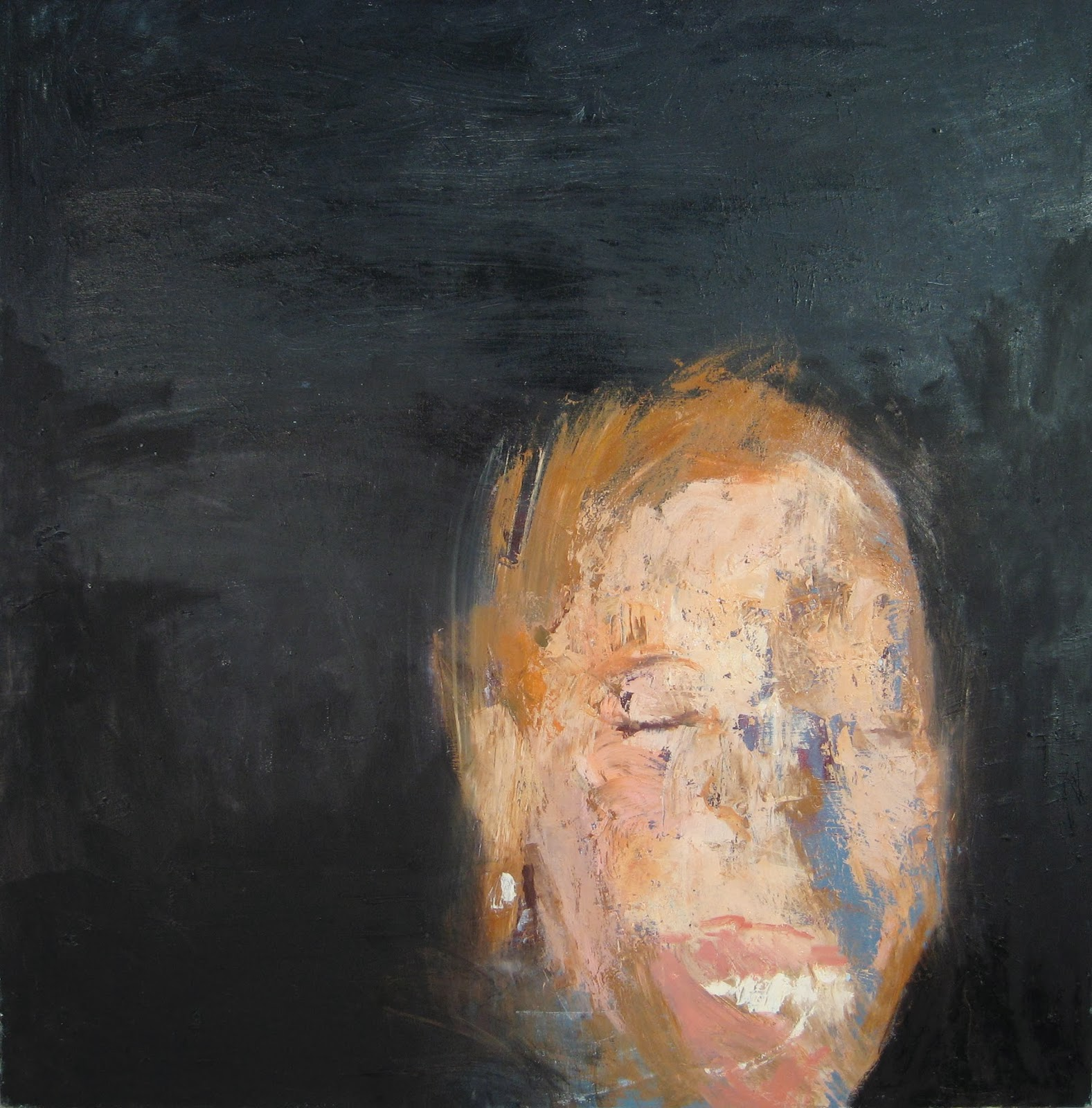girl with pearl earring, 2008, oil on canvas, 54X56"snowy november, 2012, oil on canvas, 56X56"this side now, 2012, oil on canvas, 72X84"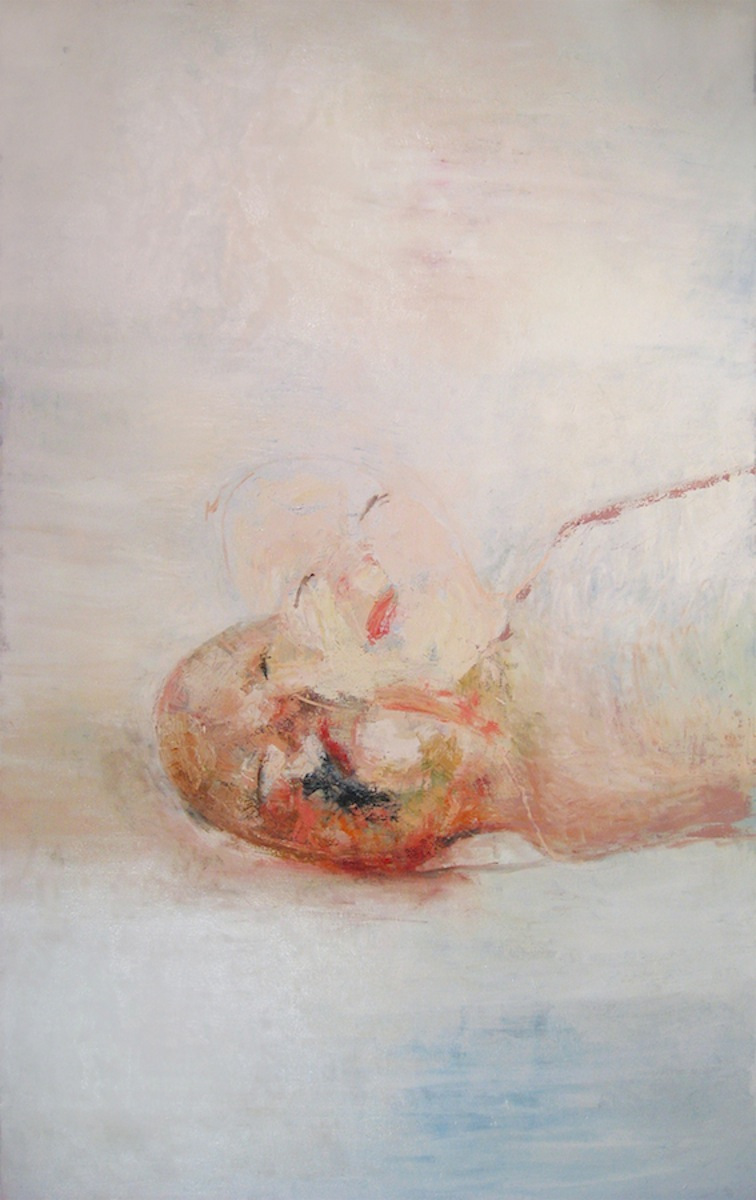baby, 2009, oil on canvas, 50X84"